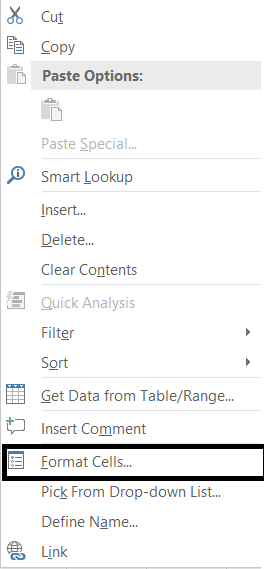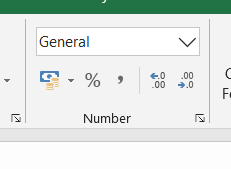PROVIDING ACCURATE, RELIABLE AND STRAIGHT TO THE POINT KNOWLEDGE ABOUT DIFFERENT IT TOOLS EXPLAINED WITH PRACTICAL EXAMPLES

# HOW TO CHANGE NUMBER FORMAT OR CELL FORMAT IN EXCEL?

## INTRODUCTION

If you are an EXCEL USER, you must be acquainted with this word “NUMBER FORMAT” or sometimes we call it as “CELL FORMAT” i.e. the format of the cell or in layman language , specifying the type of content in the cell. If we go for a definition

“NUMBER FORMATS ARE THE TYPES OF DATA ANY CELL IS CONTAINING OR IT CAN BE STATED THAT THE WAY, IN WHICH THE VALUE IS STORED IN THE CELL. E.G. STRING, NUMBER, TEXT, DATE, CURRENCY ETC.” IT HELPS EXCEL TO CALCULATE WITH ACCURACY FOR THE USER.

So rephrasing the definition, suppose a cell is containing time and we don’t know that the cell is storing time. We add 10 to that cell. So do you think that the result would be accurate or anywhere near right?? The answer is NO!!!

Unless until we know what is contained in the cell , we can never be sure about the type of operation which we can apply on that particular cell.

This topic is very important, if you plan to even use EXCEL at a BASIC LEVEL. And if you plan to master it, you should know how to use the FORMAT OF THE CELL as a weapon to kill your problems.

YES!! the format of the cell is so important that many big problems are just converted into kindergarten problems if we choose the appropriate format for the cell.

Let us see what is there in the cell format.

In this article, we’ll learn about the procedure of changing the CELL FORMAT or NUMBER FORMAT or NUMBER TYPE, whatever you call it,  of the cell and learn about the different types of cell formats present in the MICROSOFT EXCEL in detail.

## BUTTON LOCATION OF DATA TYPE CHANGE IN EXCEL

The NUMBER FORMAT button is located under the HOME TAB in NUMBER group.

Or,

On the other hand, we can even get it by RIGHT CLICKING on the concerned cell and choosing FORMAT CELL

.## TYPES OF NUMBER FORMATS IN EXCEL

The following formats of the cell are found in EXCEL.
•  GENERAL
• NUMBER
• CURRENCY
• ACCOUNTING
• DATE
• TIME
• PERCENTAGE
• FRACTION
• SCIENTIFIC
• TEXT
• SPECIAL
• CUSTOM
We’ll visit each of these options one by one in detail.

## DETAILED EXPLANATION OF FORMATS

#### GENERAL NUMBER FORMAT

This is the default format of the EXCEL CELLS which it applies whenever we type anything in the cell. The data will be displayed the same way we type. We type the text, it would show the text, we type the number ,it would show the number.

But this format is not so simple. It plays tricks.

If you type the text, it would remain a general format but would behave as a text cell and if entered a number it would behave as a numbered cell. It would round the decimal parts if the number is lengthy and it’ll go to scientific notation too after 12 digits. [Scientific notation is in the format 1.12 E^12]. If you just type the % , it’ll convert itself to percentage type, similarly if time, it’ll go to time type, if date then date etc.

WHEN TO USE GENERAL FORMAT:

IF-

• we are creating a normal report.
• We are using text and simple numbers with decimals.
• we do not have any special requirements like time, currency, scientific notation or any special format of digits etc.

In these cases , we use GENERAL FORMAT for the cells.

### NUMBER- NUMBER FORMAT​

The NUMBER FORMAT is used when we specifically declare any cell to contain numbers. It gives us additional option to choose the decimal places and if we want to use the separator after every 1000 or not.

It also gives the option to choose the way we want to show negative numbers.

We can show negative numbers by a minus (-) sign or by red color or both. This option is given below and shown in the picture below.

WHEN TO USE NUMBER-NUMBER FORMAT:

• When we need to do some numerical expressions.
• When we want to show the negative numbers in different color.

### CURRENCY NUMBER FORMAT​

Used for general monetary (currency) values and displays the default currency symbol with numbers. You can specify the number of decimal places that you want to use and how you want to display negative numbers.

WHEN TO USE CURRENCY NUMBER FORMAT:

• When we are dealing with currency and want the currency symbol to be used with the figure.
• When need to use currency with decimals.
*As we know currency can’t be negative, we can use red color for the debits and normal color for credits.

### ACCOUNTING NUMBER FORMAT​

The options are available to choose the currency of the country and number of decimal digits for the figures.

WHEN SHOULD WE USE ACCOUNTING NUMBER FORMAT:

•  For some accounting job, column holding the currency.
• Decimal places can be given to the currency.
DIFFERENCE BETWEEN CURRENCY AND ACCOUNTING NUMBER FORMAT:
If we are creating a balance sheet and showing the credit and debit. We have an additional option in the currency number format for showing the negative numbers with a color. As the currency can’t be negative, we can use that notation easily. It is not present in the accounting number format.

### DATE NUMBER FORMAT​

One of the very tricky and important format.

EXCEL stores DATE as a serial number counted from JAN 1 1900. And converts this serial number into different types of date formats which we can choose from the given list.

Choose any format from the given list.

Sometimes a problem occurs when we can’t find the format of our choice. Mostly this is due to the location settings. Choose the correct location and you would find your desired format of date.

We can choose the location and EXCEL would populate different formats available from which we can choose our format.

WHEN SHOULD WE USE DATE NUMBER FORMAT

Whenever we are working with the dates, which is going to be quite often, we should use date format for the ease which is provided by this format while using dates in our calculations.

### TIME NUMBER FORMAT​

Again very tricky format and very important. The time is stored in excel as the decimal part of the number. For example 0.5 means 12 noon and so on. There are different formats of time from which we can choose how to show the time.

Choose the time format from the given list.

If somehow, can’t find the option of your choice, choose your location and the options will change. Now choose the desired one.

WHEN SHOULD WE USE TIME NUMBER FORMAT:

We should use time number format when we are working with the time in reports. It is advisable to understand the time format and use it efficiently and it’ll make our job extremely easy.

### PERCENTAGE NUMBER FORMAT​

It simply converts the value to percentage by multiplying by 100 and putting percentage symbol with it. (%). It gives the option of choosing decimal places too.

WHEN SHOULD WE USE USE PERCENTAGE NUMBER FORMAT:

Its very obvious, when we are dealing with percentages.

*There is another way of setting the format as PERCENTAGES. Just type the number and put % sign with this. It’ll convert the cell to percentage automatically.

### FRACTION NUMBER TYPE

Displays a number as a fraction, according to the type of fraction that you specify.

Choose the fraction type from the options given below.

WHEN SHOULD WE USE FRACTION NUMBER FORMAT:

Normally, when we enter any fraction in the cell e.g. 115/215, the division takes place and the result in decimals is shown in the cell. Now if we explicitly want the fraction to be stored in the number, we should make use of this format.

### SCIENTIFIC NUMBER TYPE

Displays the number in the scientific notation i.e. Number x 10 ^ 13 or like that. The decimal parts in the number can be set, rest will come with the exponent. E here will count for 10 as we are working in the decimal system.

Although when any number goes beyond 12 digits even in GENERAL FORMAT, the number itself converts to the exponential form.

Choose the number of decimals from the given option.

WHEN SHOULD WE USE SCIENTIFIC NUMBER FORMAT:

Its upto us. If the report needs the scientific notation , we can make use of this format.

Sometimes the figures go very large, and it is not advisable to use the figures as we need to count the digits, in such cases too, we can use it.

e.g. \$1231231231231231.

Now , one would need to keep counting the digits before he can read it.

We can also write it as \$1.23 x10 ^ 15 which is 1.23 quadrillion.

### TEXT NUMBER TYPE

Text format treats everything as a text. Numbers, decimals, anything whatever goes is a text and doesn’t operate any mathematical formula on this . It’ll just respond to text functions.

WHEN SHOULD WE USE TEXT NUMBER FORMAT:

When we need to enter text in the cell.

### SPECIAL NUMBER TYPE

Displays any special type like social security number or zip code etc.

### CUSTOM NUMBER TYPE

This is the most important and versatile kind of number type.

It is the final resort for the number type problems. If our problems are not being sorted out by any of the listed formats, we should go for the custom format.

We can create a custom format with the help of this option. The picture shows the steps to make your own custom format.

STEPS:

1. Search the desired format in the list. If not available type your custom format in the TYPE field as

shown in the picture.

2. Check the sample before finalizing.

3. Click OK and custom format is ready.

WHEN SHOULD WE USE CUSTOM NUMBER FORMAT:

It is extremely useful format choice.

Phone numbers, zip codes, any other special number etc.

## PROCEDURE TO CHANGE NUMBER FORMAT/DATA TYPES IN EXCEL

STEPS:

• Select the cell or group of cells whose format we want to change.
• Right Click the selection area.
• Choose FORMAT CELLS.
• Choose the format from the list.
• The format is converted to the selected one.
Another faster way is there for easy formatting of cells.
• Select the cell or cells whose format we want to change.
• Go to HOME TAB > NUMBER CELLS
• A drop down containing the major cell formats is present there.
•• Choose the desired format.
• The cells are converted to desired formats.

• ## CHANGE NUMBER FORMAT IN EXCEL, number format excel, data type excel, how to write percentage in excel, how to write fraction in excel, how to type time in excel, how to type date in excel, custom number format excel

LEARN

• EXCEL
• JAVASCRIPT
• MORE TO COME…

Encourage us by following at …

If you have any queries or suggestions or any problem which you would like us to solve for you, kindly mail at

[email protected]